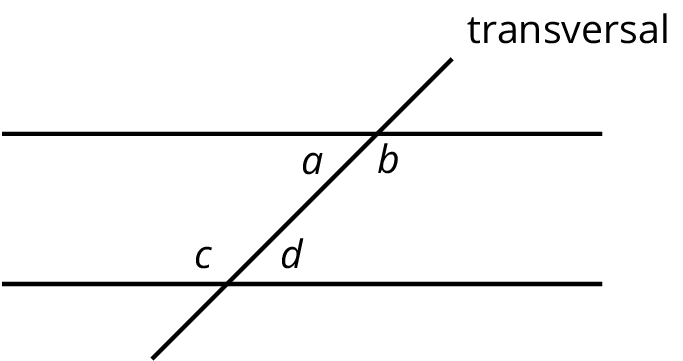# Lesson 14

Parallel Lines and the Angles in a Triangle

Let’s see why the angles in a triangle add to 180 degrees.

### 14.1: True or False: Computational Relationships

Is each equation true or false?

$$62-28= 60-30$$

$$3\boldcdot \text{-}8= (2\boldcdot \text{-}8) - 8$$

$$\dfrac {16}{\text{-}2} + \dfrac{24}{\text{-}2} = \dfrac{40}{\text{-}2}$$

### 14.2: Angle Plus Two

Consider triangle $$ABC$$. Select the Midpoint tool and click on two points or a segment to find the midpoint.

1. Rotate triangle $$ABC$$ $$180^\circ$$ around the midpoint of side $$AC$$. Right click on the point and select Rename to label the new vertex $$D$$.

2. Rotate triangle $$ABC$$ $$180^\circ$$ around the midpoint of side $$AB$$. Right click on the point and select Rename to label the new vertex $$E$$.

3. Look at angles $$EAB$$, $$BAC$$, and $$CAD$$. Without measuring, write what you think is the sum of the measures of these angles. Explain or show your reasoning.

4. Is the measure of angle $$EAB$$ equal to the measure of any angle in triangle $$ABC$$? If so, which one? If not, how do you know?

5. Is the measure of angle $$CAD$$ equal to the measure of any angle in triangle $$ABC$$? If so, which one? If not, how do you know?

6. What is the sum of the measures of angles $$ABC$$, $$BAC$$, and $$ACB$$?

### 14.3: Every Triangle in the World

Here is $$\triangle ABC$$. Line $$DE$$ is parallel to line $$AC$$.

1. What is $$m{\angle DBA} + b + m{\angle CBE}$$? Explain how you know.

2. Use your answer to explain why $$a + b + c = 180$$.
3. Explain why your argument will work for any triangle: that is, explain why the sum of the angle measures in any triangle is $$180^\circ$$.

1. Using a ruler, create a few quadrilaterals. Use a protractor to measure the four angles inside the quadrilateral. What is the sum of these four angle measures?

2. Come up with an explanation for why anything you notice must be true (hint: draw one diagonal in each quadrilateral).

### 14.4: Four Triangles Revisited

This diagram shows a square $$BDFH$$ that has been made by images of triangle $$ABC$$ under rigid transformations.

Given that angle $$BAC$$ measures 53 degrees, find as many other angle measures as you can.

### Summary

Using parallel lines and rotations, we can understand why the angles in a triangle always add to $$180^\circ$$. Here is triangle $$ABC$$. Line $$DE$$ is parallel to $$AC$$ and contains $$B$$.

A 180 degree rotation of triangle $$ABC$$ around the midpoint of $$AB$$ interchanges angles $$A$$ and $$DBA$$ so they have the same measure: in the picture these angles are marked as $$x^\circ$$. A 180 degree rotation of triangle $$ABC$$ around the midpoint of $$BC$$ interchanges angles $$C$$ and $$CBE$$ so they have the same measure: in the picture, these angles are marked as $$z^\circ$$. Also, $$DBE$$ is a straight line because 180 degree rotations take lines to parallel lines. So the three angles with vertex $$B$$ make a line and they add up to $$180^\circ$$ ($$x + y + z = 180$$). But $$x, y, z$$ are the measures of the three angles in $$\triangle ABC$$ so the sum of the angles in a triangle is always $$180^\circ$$!

### Glossary Entries

• alternate interior angles

Interior angles are angles that are made by a transversal crossing two parallel lines. They are the angles that lie between the parallel lines, not outside them.

If two interior angles lie on opposite sides of the transversal they are called alternate interior angles.

In the figure, $$a$$ and $$d$$ are alternate interior angles, and $$b$$ and $$c$$ are also alternate interior angles.• complementary

Complementary angles have measures that add up to 90 degrees.

For example, a $$15^\circ$$ angle and a $$75^\circ$$ angle are complementary.

• straight angle

A straight angle is an angle that forms a straight line. It measures 180 degrees.

• supplementary

Supplementary angles have measures that add up to 180 degrees.

For example, a $$15^\circ$$ angle and a $$165^\circ$$ angle are supplementary.

This diagram shows a transversal line $$k$$ intersecting parallel lines $$m$$ and $$\ell$$.